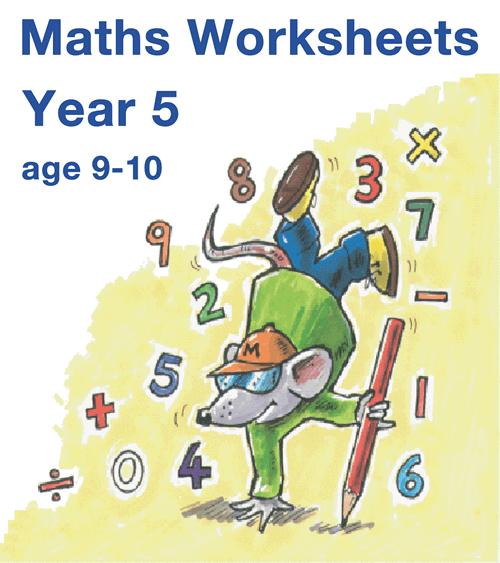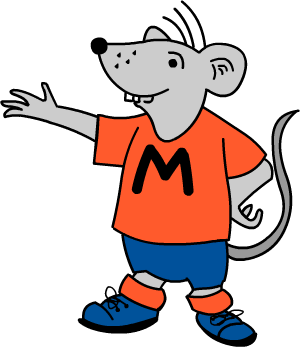# Year 5 Maths Worksheets

Enjoy teaching the new 2014 Programme of Study with this superb collection of maths worksheets for Year 5 (9‐10 year olds). The vast range of MathSphere worksheets gives you plenty of choice in selecting the right resources to ensure understanding and progression for every child.

## The Very Best Year 5 Maths Worksheets

There are plenty of challenges in the new curriculum, including working with very large numbers, Roman numerals, decimals and addition and subtraction of fractions. We have carefully updated all our resources to cover these new challenges.

• Updated for the very latest statutory requirements with many new pages added
• Answers included to make marking simple
• Concept pages clearly explain the ideas being taught
• Over 800 high quality pages of questions and answers
• Single user licence for teachers or parents (school site licence upgrade available)
• Thousands of teachers have been successfully using MathSphere for over 15 years## Try Some Free Sample Worksheets

To give you a better idea of the worksheets available we have provided a selection of free modules for Year 5.

Please download these samples, print them out and use at home, or photocopy and use in the classroom.11 Pages

#### Revise Estimating & Approximating

Estimating on number lines and plenty of practical ideas to challenge children.6 Pages

#### Roman Numerals

How to read Roman numerals up to thousands.12 Pages

#### Square, Prime, Factors & Multiples

Plenty of activities using these four types of numbers.

## List of modules

It is impossible to describe in a single page the massive amount of high quality maths contained in this one product.

To give you some idea, have a look at the list of all modules:

### Number and Place Value 92 pages

 Read and write whole numbers. Partition. 21 Revise comparing and ordering numbers. 10 Count in powers of ten. 4 Extend number sequences. 12 Recognise and order negative numbers. 13 Solve 'real life' problems 8 Rounding numbers. 7 Revise estimating and approximating. 11 Roman Numerals. 6

### Multiplication and Division 176 pages

 Revise understanding multiplication. 13 Revise understanding division. 12 Revise multiplying and dividing by 10 and 100. 12 More multiplying and dividing by 10 and 100. Double and halve. 13 Multiply and divide by 10, 100 and 1000. 9 Double and halve. Use factors to help calculate. 18 Use knowledge of tables to calculate mentally. 17 Square numbers, prime numbers, factors and multiples. 12 Revise and refine written methods of multiplication. 11 Short multiplication. 5 Written multiplication of decimals. 8 Long multiplication. 8 More long multiplication. 8 Revise written methods for division. 8 Revise short division. 7 Divide a 4-digit number by a 1-digit number. 4 Square and cube numbers, square roots and prime factors. 11

### Measurement 104 pages

 Units to measure length, mass or capacity. 20 Standard metric units of measurement. 10 Metric and Imperial. 5 Read scales, record, estimate and measure. 16 Perimeter and area. 18 Estimating and measuring time. 9 Solve problems involving time. 8 Solve problems involving length, mass or capacity. 7 Solve problems involving money. 8 Investigate cuboids. 3

### Statistics 46 pages

 Collect and organise data. Use tables, graphs and charts (1) 11 Further data handling. Use median and mode. 10 Interpreting graphs, tables and charts. (Also Booster material) 21 Two dice investigation. 4

### Fractions 83 pages

 Recognise equivalent fractions. Order fractions. 13 Find fractions of numbers or quantities. 6 Adding fractions. 7 Subtracting fractions. 7 Multiplying fractions. 5 Round decimals. Equivalence between decimals and fractions. 16 Order decimals: thousandths. 9 Understand percentages. 13 Find percentages of numbers or quantities. 7

### Geometry 61 pages

 Describe and classify 3D and 2D shapes. 11 Estimate, draw and measure angles. 10 Making shapes and patterns. 7 Recognise position and direction. Use co-ordinates. 13 Reflective symmetry. Reflections and translations. 15 Tessellation investigation. 5

### Using and Applying Mathematics 58 pages

 Checking results of calculations. 11 Use a calculator. 9 Probability: using the language of chance or likelihood. 7 Maths vocabulary games. 8 Investigate zero. 4 Investigate 10. 4 Investigate products. 3 Triangle numbers. 12

### Mental arithmetic 75 pages

 Autumn term. 25 Spring term. 25 Summer term. 25

### Quick Details

• Over 800 worksheets